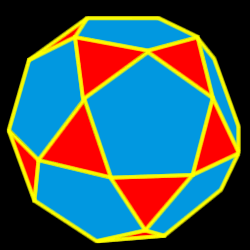# Icosidodecahedron

Properties of the icosidodecahedron: Number of faces, edges and dihedral angle measure

The icosidodecahedron is created by either truncating (cutting off) the dodecahedron one half of the way into each side or truncating the icosahedron one half of the way into each side.32 total faces:
20 equilateral triangles and 12 regular pentagons

30 vertices:
2 triangles and 2 pentagons

60 edges

Dihedral angles:  142 degrees, 37 minutes